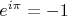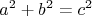# texdrive

Sometimes, a formula says more than a 1000 words...texdrive is an emacs minor-mode for adding mathematical equations to html-pages; things like Euler's, Pythagorasand so on... It's a replacement for a little tool called WebTex that I wrote a few years ago.

### news

• [2009-02-22] Version 0.2 released. Minor fixes.

### usage

Suppose you want to include the famous Bayes' Theorem. Simply type:
  M-x texdrive-insert-formula
Formula: $P(A|B) = \frac{P(B|A)P(A)}{P(B|A)P(A) + P(B|\overline{A})P(\overline{A})}$
Title: bayes-theorem

Et voilà; the following is inserted:
  <img src="bayes-theorem.png" title="bayes-theorem"
class="texdrive-formula" name="$P(A|B) = \frac{P(B|A)P(A)}{P(B|A)P(A) + P(B|\overline{A})P(\overline{A})}$"
border="0">
Now, all we need to do is texdrive-generate-images-from-html RET, and the corresponding image will be generated:### requirements

texdrive requires LaTeX and Imagemagick. In Debian/Ubuntu, you can install the packages texlive and imagemagick. LaTeX (texlive) is a huge package, but of course it can do a lot more than render formulae for texdrive.

  (require 'texdrive)
to your .emacs. Then, to enable the texdrive minor mode:
  (texdrive-mode)
This will enable two keybindings:
• C-c TAB f for texdrive-insert-formula
• C-c TAB g for texdrive-generate-images
These keybindings fit in with the html-helper-mode keybindings. There als some variables you might want to customize:
• texdrive-img-path - the (relative) path where images are stored; if you store images in some images/ directory on your webserver, you could set texdrive-image-path to that. Default is "";
• texdrive-tmp-path - determines where temporary files are stored; by default this is in /tmp, but you can set it to somewhere else if you don't trust the other users on your system.
There are some other variables that you probably don't want to change; but see the texdrive.el source file for details.

texdrive is published under the terms of the GNU General Public License (GPL), version 3 or later.

### anticipated questions

• Why is it called 'texdrive'? I don't know; have any better ideas?
• What kind of LaTeX environment is texdrive using for the formulae? It's using the single-$math environment by default; if you want to use the '$$' environment, simply put '$' at the beginning/end of your formula;
• I don't like the Computer Modern font that texdrive is using; how can I change it? At this moment, you cannot change it without changing the texdrive source code, but it's pretty easy. Search for '\\usepackage' and add your favourite fontpackage there, as outlined in A Survey of Free Math Fonts for TeX and LaTeX;
• I want to use texdrive to generate images from formulae outside emacs. How can I do that? Use emacs in batch-mode; create a shell script texdrive.sh like:
  #!/bin/sh
emacs -batch -nw --eval="(load-library \"~/.elisp/texdrive.el\")" --eval="(texdrive-create-png \"$1\" \"$2\")" 2>&1 | grep -v 'Loading\|Skipping'

Now, use
  \$ texdrive.sh abc 'a^2 + b^2 = c^2'
to create abc.png. (Note: you'll probably need to update the path to 'texdrive.el'.)